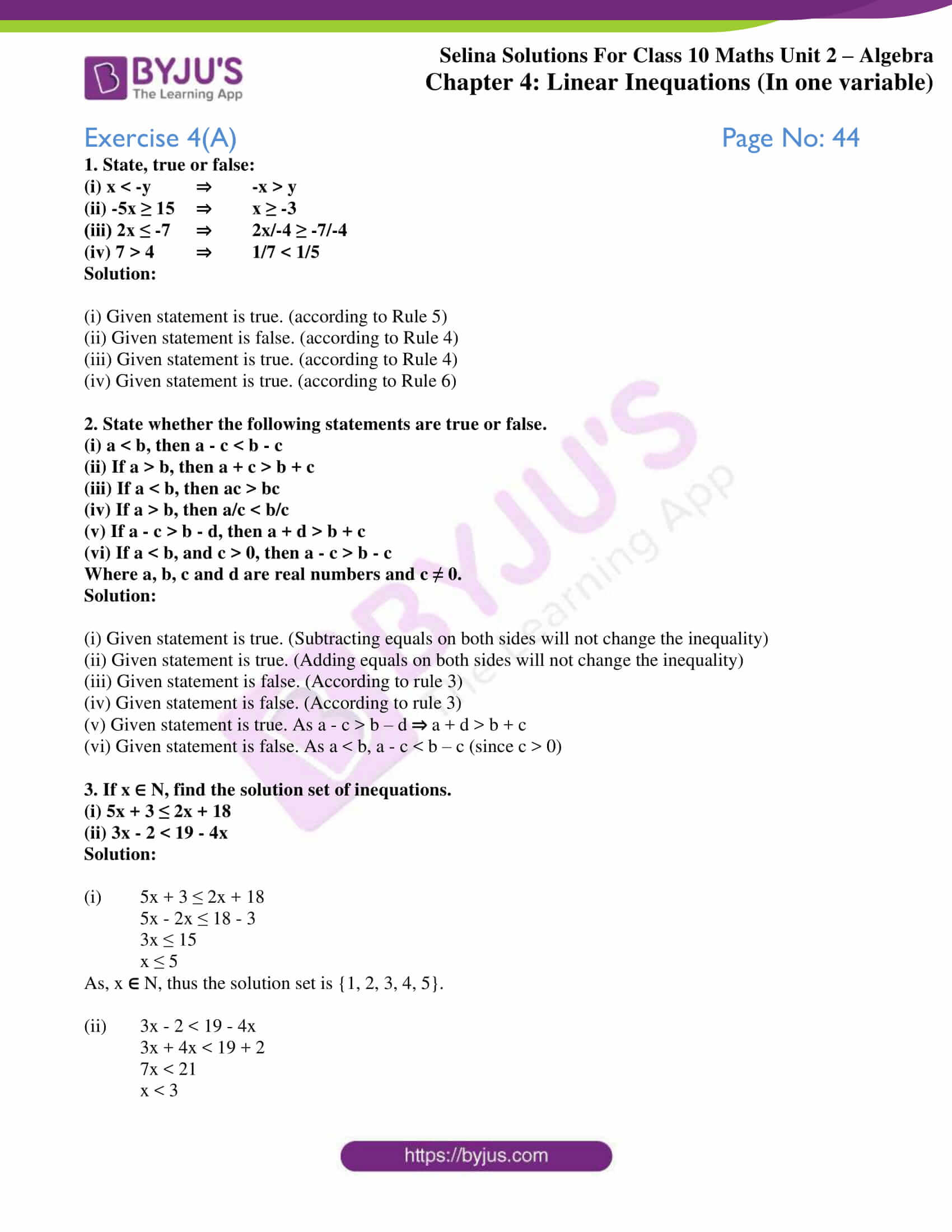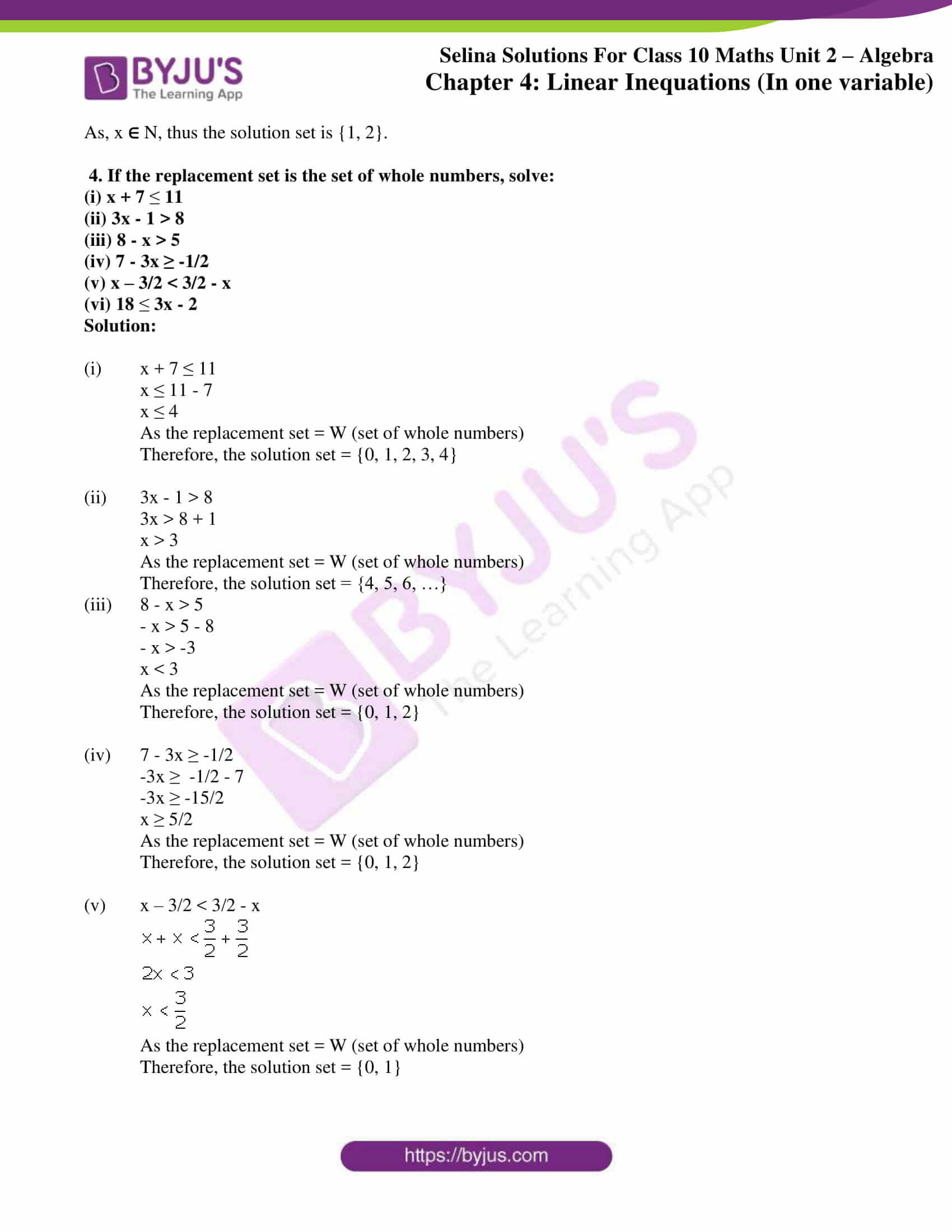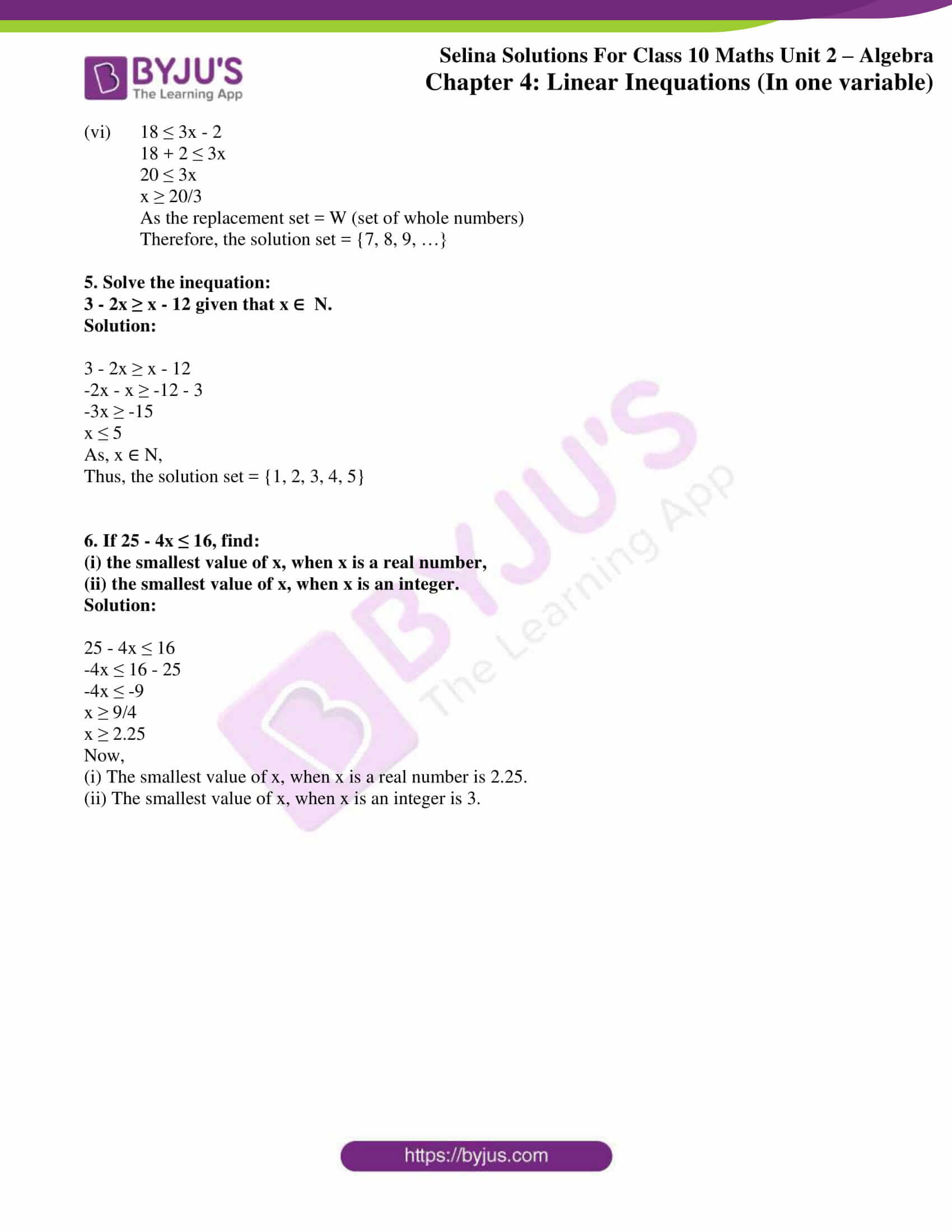# Selina Solutions Concise Maths Class 10 Chapter 4 Linear Inequations (In one variable) Exercise 4(A)

Solving linear inequations in one variable algebraically is done by following certain rules. The understanding of the replacement set and the solution set is made clear when solving problems of this exercise. The Selina Solutions for Class 10 Maths is a powerful tool for students to do any quick reference or doubt clearance anytime. Students can now get the Concise Selina Solutions for Class 10 Maths Chapter 4 Linear Inequations (In one variable) Exercise 4(A) PDF in the links given below.

## Selina Solutions Concise Maths Class 10 Chapter 4 Linear Inequations (In one variable) Exercise 4(A) Download PDF### Access Selina Solutions Concise Maths Class 10 Chapter 4 Linear Inequations (In one variable) Exercise 4(A)

1. State, true or false:

(i) x < -y ⇒ -x > y

(ii) -5x ≥ 15 ⇒ x ≥ -3

(iii) 2x ≤ -7 ⇒ 2x/-4 ≥ -7/-4

(iv) 7 > 4 ⇒ 1/7 < 1/5

Solution:

(i) Given statement is true. (according to Rule 5)

(ii) Given statement is false. (according to Rule 4)

(iii) Given statement is true. (according to Rule 4)

(iv) Given statement is true. (according to Rule 6)

2. State whether the following statements are true or false.

(i) a < b, then a – c < b – c

(ii) If a > b, then a + c > b + c

(iii) If a < b, then ac > bc

(iv) If a > b, then a/c < b/c

(v) If a – c > b – d, then a + d > b + c

(vi) If a < b, and c > 0, then a – c > b – c

Where a, b, c and d are real numbers and c ≠ 0.

Solution:

(i) Given statement is true. (Subtracting equals on both sides will not change the inequality)

(ii) Given statement is true. (Adding equals on both sides will not change the inequality)

(iii) Given statement is false. (According to rule 3)

(iv) Given statement is false. (According to rule 3)

(v) Given statement is true. As a – c > b – d a + d > b + c

(vi) Given statement is false. As a < b, a – c < b – c (since c > 0)

3. If x ∈ N, find the solution set of inequations.

(i) 5x + 3 ≤ 2x + 18

(ii) 3x – 2 < 19 – 4x

Solution:

(i) 5x + 3 ≤ 2x + 18

5x – 2x ≤ 18 – 3

3x ≤ 15

x ≤ 5

As, x  N, thus the solution set is {1, 2, 3, 4, 5}.

(ii) 3x – 2 < 19 – 4x

3x + 4x < 19 + 2

7x < 21

x < 3

As, x  N, thus the solution set is {1, 2}.

4. If the replacement set is the set of whole numbers, solve:

(i) x + 7  11

(ii) 3x – 1 > 8

(iii) 8 – x > 5

(iv) 7 – 3x ≥ -1/2

(v) x – 3/2 < 3/2 – x

(vi) 18  3x – 2

Solution:

(i) x + 7 ≤ 11

x ≤ 11 – 7

x ≤ 4

As the replacement set = W (set of whole numbers)

Therefore, the solution set = {0, 1, 2, 3, 4}

(ii) 3x – 1 > 8

3x > 8 + 1

x > 3

As the replacement set = W (set of whole numbers)

Therefore, the solution set = {4, 5, 6, …}

(iii) 8 – x > 5

– x > 5 – 8

– x > -3

x < 3

As the replacement set = W (set of whole numbers)

Therefore, the solution set = {0, 1, 2}

(iv) 7 – 3x ≥ -1/2

-3x ≥  -1/2 – 7

-3x ≥ -15/2

x ≥ 5/2

As the replacement set = W (set of whole numbers)

Therefore, the solution set = {0, 1, 2}

(v)  x – 3/2 < 3/2 – x

x + x < 3/2 + 3/2

2x < 3

x < 3/2

As the replacement set = W (set of whole numbers)

Therefore, the solution set = {0, 1}

(vi) 18 ≤ 3x – 2

18 + 2 ≤ 3x

20 ≤ 3x

x ≥ 20/3

As the replacement set = W (set of whole numbers)

Therefore, the solution set = {7, 8, 9, …}

5. Solve the inequation:

3 – 2x ≥ x – 12 given that x ∈  N.

Solution:

3 – 2x ≥ x – 12

-2x – x ≥ -12 – 3

-3x ≥ -15

x ≤ 5

As, x ∈ N,

Thus, the solution set = {1, 2, 3, 4, 5}

6. If 25 – 4x ≤ 16, find:

(i) the smallest value of x, when x is a real number,

(ii) the smallest value of x, when x is an integer.

Solution:

25 – 4x ≤ 16

-4x ≤ 16 – 25

-4x ≤ -9

x ≥ 9/4

x ≥ 2.25

Now,

(i) The smallest value of x, when x is a real number is 2.25.

(ii) The smallest value of x, when x is an integer is 3.